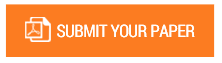A Rigorous Reformulation of Einstein derivation of the special relativity

Authors : Dr. Ismail Abbas

Volume/Issue : Volume 7 - 2022, Issue 2 - February

Scribd : https://bit.ly/3sEh8QB

DOI : https://doi.org/10.5281/zenodo.6324421

It is true that the conclusions of Einstein SR in 1905 are perfect and incontestable but on the other hand it is also real that his derivation is doubtful and fundamentally fluid or not rigorous on certain physical points. However, while each of his 5 assumptions is impossible to dispute or prove wrong, we assume that not all of them are essential to proving Einstein SR. To be precise, we assume that at least one of them is inaccurate. Namely, combining the relativistic mass transformation with Newton's law of motion which is highly contested in modern quantum mechanical theory, but which was key to arriving at Einstein's own derivation of his famous mass-energy equivalence relationship Einstein's derivation of the relativistic mass transformation using Newton's law of motion to derive the mass-energy equivalence relation inaccurate. Moreover, its derivation from the Lorentz transformation LT is useless because LT itself is a universal law of physics that applies to EMW propagation as well as the partial differential equation of diffusion and any physical phenomenon in 4D spacetime. It doesn't need a bypass

#### CALL FOR PAPERS

Paper Submission Last Date
31 - May - 2023